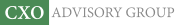Objective research to aid investing decisions

#### Value Investing Strategy (Strategy Overview)

Allocations for January 2021 (Final)
Cash TLT LQD SPY

#### Momentum Investing Strategy (Strategy Overview)

Allocations for January 2021 (Final)
1st ETF 2nd ETF 3rd ETF

# Stock Size and Excess Stock Portfolio Growth

| | Posted in: Size Effect

Why do simple stock portfolios such as equal weighting and random weighting beat market capitalization weighting over the long run? In their June 2018 paper entitled "Diversification, Volatility, and Surprising Alpha", Adrian Banner, Robert Fernholz, Vassilios Papathanakos, Johannes Ruf and David Schofield tackle this question by decomposing expected stock portfolio log-return into average growth rate and excess growth rate (EGR). They focus on average log-return because, unlike arithmetic and geometric averages, it is an unbiased estimator of long-term performance. They apply two formulas derived in prior work to estimate portfolio log-returns:

1. Expected portfolio log-return = weighted average stock log-return + EGR
2. EGR = (weighted average stock return variance - portfolio return variance)/2

They apply these formulas to the following five portfolios, each consisting of monthly overlapping sub-portfolios formed from the 1,000 U.S. stocks with the (each day) largest market capitalizations and rebalanced annually with stock weights normalized to a sum of one:

1. Capitalization-weighted (CW) - stock weights are proportional to their respective market capitalizations.
2. Equal-weighted (EW) - weight of each stock is 1/1000.
3. Large-overweighted (LO) - stock weights are proportional to the square of their respective market capitalizations.
4. Random-weighted (RW) - stock weights are proportional to random values between zero and one (median of 1,000 trials).
5. Inverse random-weighted (IRW) - stock weights are proportional to the reciprocals of random values between zero and one (median of 1,000 trials).

EGR quantifies the extent to which portfolio volatility is less than constituent stock volatilities and is always positive for long-only portfolios. Higher constituent stock volatilities generate higher portfolio EGRs. Using daily prices for the 1,000 U.S. stocks with the largest market capitalizations each day during 1964 through 2012 (5,384 distinct stocks over 49 years), they find that: